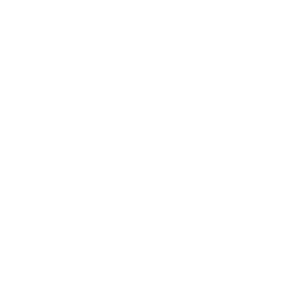## What is Quantification Anyway?

Bioanalytical analysis is a fundamental tool for the pharmacokineticist. The results of a bioanalysis are the source data for all pharmacokinetic work. Thus a clear understanding of the methodologies and challenges associated with the bioanalytical analysis science is of great benefit to a pharmacokineticist. As an example, I was recently working on the development of a new compound. Several clinical trials had been run before I became involved in the project, and I was asked to take the molecule from its current state to registration (eg, NDA with the FDA). As I began to review the pharmacokinetic data from the previous four clinical trials, I started to ask questions about the bioanalytical method being used to measure plasma concentrations. I discovered, that the drug was not stable in plasma, and there was rapid degradation after just a few minutes of time at room temperature. I also discovered that our pre-clinical group had begun to use a stabilizer in plasma collection tubes to prevent this degradation. However, that same stabilizer was not being used in the clinical setting. Furthermore, all of the projections of drug exposure for future studies were based off analyses of plasma samples without the stabilizer.

Clearly, the human exposure data was compromised, and potentially unreliable. We decided to conduct a few simple in vitro experiments and determined that the stabilizer was needed for human plasma samples also. We then used that stabilizer in an upcoming clinical pharmacology study and the results showed that the previous exposure estimates (without stabilizer) were off by as much as 50%! Furthermore, a projected difference in exposure between patients and healthy volunteers from the older studies turned out to be an artifact due to the poor bioanalysis technique used. Once we corrected the bioanalysis methodology, we were able to obtain high quality data with lower variability and more accuracy.

### Limit of quantification (LOQ)

The limit of quantification is defines as the lowest concentration which can be determined with a given analytical assay with the required precision and accuracy. In most cases the precision and accuracy must be ± 20%, but sometimes it is ±15%. This means that the actual value can be within 15-20% of the reported value. If the LOQ is reported as 1.00 ng/mL, then the actual concentration can be between 0.80 ng/mL and 1.20 ng/mL (assumes ±20%). Values below the limit of quantification (abbreviated as BLQ or BQL) are not reported as number in most bioanalysis datasets. The bioanalyst does not report the value because he/she does not have adequate confidence in the accuracy of the number to report it. (Some pharmacokineticists would like this information and the associated variability reported… but that is a separate discussion.)

### Limit of detection (LOD)

The limit of detection is the lowest concentration which can be measured analytically using prespecified criteria. Often the limit of detection is a bioanalytical response that is five times the background response in the assay. Below this limit, the bioanalytical scientist believes he/she cannot separate background noise from an actual analyte measurement.

### Upper limit of quantification (ULOQ or ULQ)

The upper limit of quantification is the highest concentration in the calibration curve which can be determined with a given analytical assay with the required precision and accuracy. In most cases the precision and accuracy must be ±15% at the high end of the calibration curve. The highest concentration is selected arbitrarily by the bioanalytical scientist. If concentrations are observed above the ULOQ, the samples can be diluted and tested within the calibration range. Often the bioanalytical scientist will try to cover at least 3 orders of magnitude (eg, 1 to 1000 ng/mL) in the analytical range to avoid having to do dilution work.

### Precision

Precision is a measure of reproducibility or repeatability of a measurement. If the same sample is measured multiple times, the analytical assay may provide slightly different values (ie, concentrations). Validated assays have an expected precision less than or equal to ±15% at all concentrations except the LOQ where precision of ±20% is acceptable. The precision can be determined by making at least 3 measurements of the same sample. The coefficient of variation (%CV) is calculated by dividing the standard deviation of the 3 measurements by the mean of the 3 measurements and multiplying by 100. Thus if the standard deviation is 4.37 ng/mL, and the mean is 49.6 ng/mL, the %CV would be$\textrm{\%CV}={\frac{4.37}{49.6}}*100=8.76\%$

### Accuracy

Accuracy is a measure of how close a measured value is to the actual (true) value. A known concentration is measured multiple times, and the analytical assay may report different values (ie, concentrations). Validated assays are expected to have an accuracy less than or equal to ±15% at all concentrations in the assay range. The accuracy can be determined for each sample, then the mean accuracy can be reported. Accuracy is calculated using the following equation$\textrm{Accuracy (\%)}={\frac{\textrm{Measurement}-\textrm{Theoretical}}{\textrm{Theoretical}}}*100$

If three measurements of a 50 ng/mL sample are 41.5, 51.2, and 47.6 ng/mL, then the mean accuracy would be -6.47% (average of -17%, 2.4%, and -4.8%), which is within the acceptable range.

Today, drug development is carried out in human subjects and animals. However, as computing power and the number of sophisticated technology platforms grow exponentially, and our knowledge of human health and disease increases, the virtualization of clinical research and development will grow steadily. Read this article to learn more.